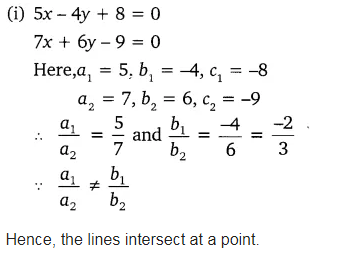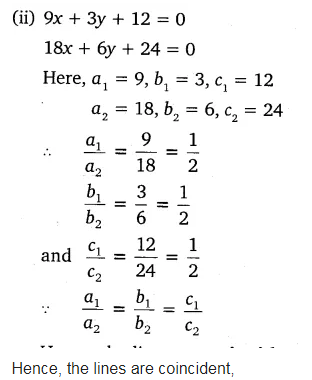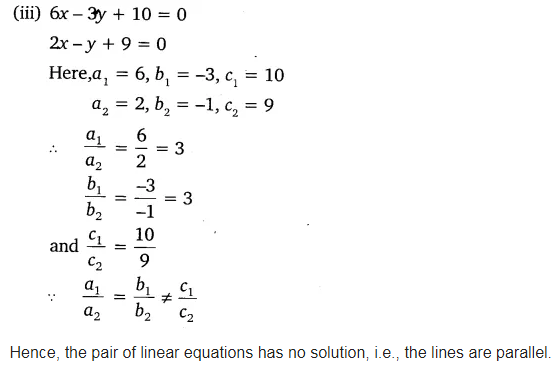# Find out whether the lines representing the following pairs of linear equations

On comparing the ratios$\frac { { a }{ 1 } }{ { a }{ 2 } }$,$\frac { { b }{ 1 } }{ { b }{ 2 } }$
and$\frac { { c }{ 1 } }{ { c }{ 2 } }$ , find out whether the lines representing the following pairs of linear equations intersect at a point, are parallel or coincident:
(i) 5x – 4y + 8 = 0, 7x + 6y – 9 = 0
(ii) 9x + 3y + 12 = 0, 18x + 6y + 24 = 0
(iii) 6x – 3y + 10 = 0, 2x -y + 9 = 0